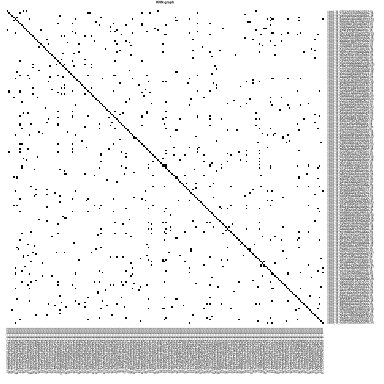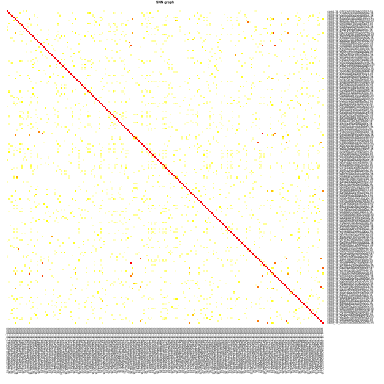# Clustering

In this tutorial we will continue the analysis of the integrated dataset. We will use the integrated PCA to perform the clustering. First we will construct a \(k\)-nearest neighbour graph in order to perform a clustering on the graph. We will also show how to perform hierarchical clustering and k-means clustering on PCA space.

Let’s first load all necessary libraries and also the integrated dataset from the previous step.

``````suppressPackageStartupMessages({
library(Seurat)
library(cowplot)
library(ggplot2)
library(pheatmap)
library(rafalib)
library(clustree)
})

## Graph clustering

The procedure of clustering on a Graph can be generalized as 3 main steps:

1. Build a kNN graph from the data

2. Prune spurious connections from kNN graph (optional step). This is a SNN graph.

3. Find groups of cells that maximizes the connections within the group compared other groups.

### Building kNN / SNN graph

The first step into graph clustering is to construct a k-nn graph, in case you don’t have one. For this, we will use the PCA space. Thus, as done for dimensionality reduction, we will use ony the top N PCA dimensions for this purpose (the same used for computing UMAP / tSNE).

As we can see above, the Seurat function `FindNeighbors` already computes both the KNN and SNN graphs, in which we can control the minimal percentage of shared neighbours to be kept. See `?FindNeighbors` for additional options.

``````# check that CCA is still the active assay
alldata@active.assay

alldata <- FindNeighbors(alldata, dims = 1:30, k.param = 60, prune.SNN = 1/15)``````
``## Computing nearest neighbor graph``
``## Computing SNN``
``````# check the names for graphs in the object.
names(alldata@graphs)``````
``````##  "CCA"
##  "CCA_nn"  "CCA_snn"``````

We can take a look at the kNN graph. It is a matrix where every connection between cells is represented as \(1\)s. This is called a unweighted graph (default in Seurat). Some cell connections can however have more importance than others, in that case the scale of the graph from \(0\) to a maximum distance. Usually, the smaller the distance, the closer two points are, and stronger is their connection. This is called a weighted graph. Both weighted and unweighted graphs are suitable for clustering, but clustering on unweighted graphs is faster for large datasets (> 100k cells).

``````pheatmap(alldata@graphs\$CCA_nn[1:200, 1:200], col = c("white", "black"), border_color = "grey90",
main = "KNN graph", legend = F, cluster_rows = F, cluster_cols = F, fontsize = 2)````````````pheatmap(alldata@graphs\$CCA_snn[1:200, 1:200], col = colorRampPalette(c("white",
"yellow", "red"))(100), border_color = "grey90", main = "SNN graph", legend = F,
cluster_rows = F, cluster_cols = F, fontsize = 2)``````### Clustering on a graph

Once the graph is built, we can now perform graph clustering. The clustering is done respective to a resolution which can be interpreted as how coarse you want your cluster to be. Higher resolution means higher number of clusters.

In Seurat, the function `FindClusters` will do a graph-based clustering using “Louvain” algorithim by default (`algorithm = 1`). TO use the leiden algorithm, you need to set it to `algorithm = 4`. See `?FindClusters` for additional options.

``````# Clustering with louvain (algorithm 1)
for (res in c(0.1, 0.25, 0.5, 1, 1.5, 2)) {
alldata <- FindClusters(alldata, graph.name = "CCA_snn", resolution = res, algorithm = 1)
}

# each time you run clustering, the data is stored in meta data columns:
# seurat_clusters - lastest results only CCA_snn_res.XX - for each different
# resolution you test.

plot_grid(ncol = 3, DimPlot(alldata, reduction = "umap", group.by = "CCA_snn_res.0.5") +
ggtitle("louvain_0.5"), DimPlot(alldata, reduction = "umap", group.by = "CCA_snn_res.1") +
ggtitle("louvain_1"), DimPlot(alldata, reduction = "umap", group.by = "CCA_snn_res.2") +
ggtitle("louvain_2"))``````